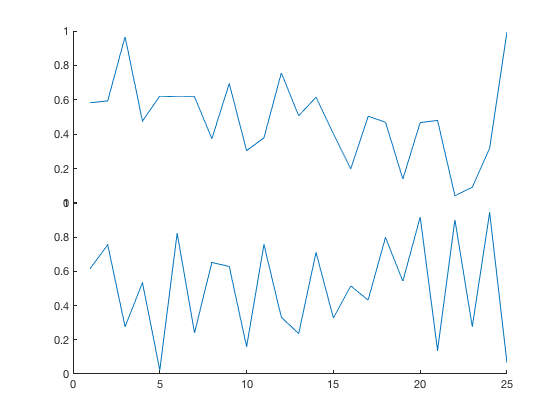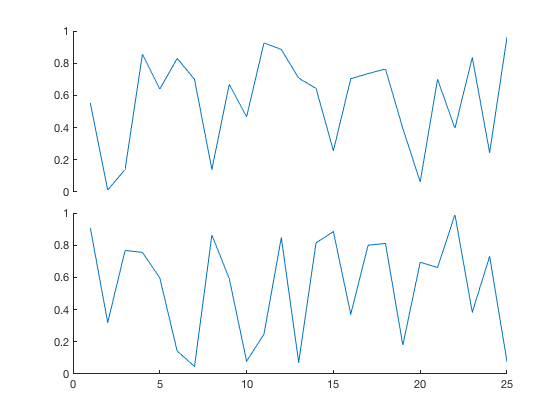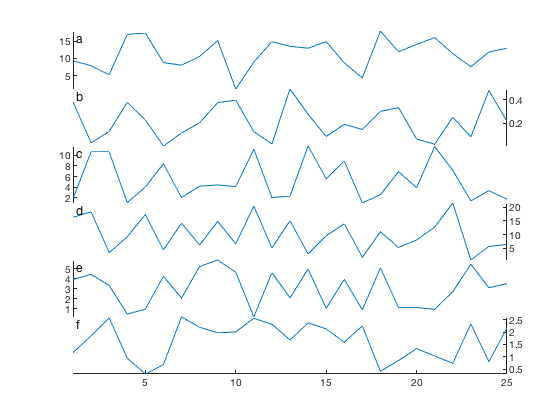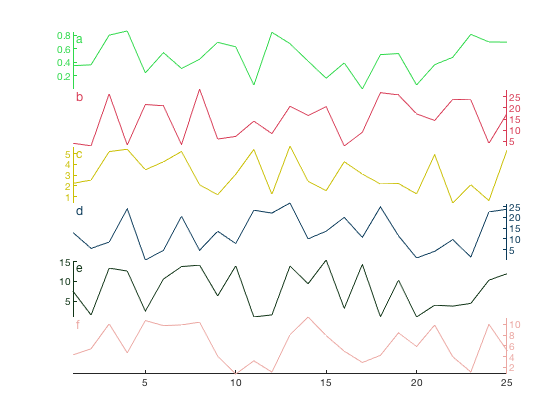# subsubplot documentation

subsubplot creates sub-axes in tiled positions.

## Syntax

```subsubplot(mm,nn,pp)
subsubplot(m,n,p,mm,nn,pp)
h = subsubplot(...)```

## Description

subsubplot(mm,nn,pp) divides the current figure into an mm-by-nn grid and creates axes in the position specified by pp. The first subplot is the first column of the first row, the second subplot is the second column of the first row, and so on. This syntax effectively fills the area that would be occupied by subplot(1,1,1).

subsubplot(m,n,p,mm,nn,pp) As above, but in this case the subplot(m,n,p) is further divided into an mm-by-nn grid, and subsubplot creates a new set of axes in the location specified by pp.

subsubplot(...,'vpad',vspace) specifies the amount of vertical spacing between each subsubplot axis, in normalized figure units on the scale of 0 to 1. The default value is 0. Setting it to 0.05, for example, separates axes by 5% of the figure height.

subsubplot(...,'hpad',hspace) specifies the amount of horizontal spacing between each subplot axis, in normalized figure units on the scale of 0 to 1. The default value is 0. Setting it to 0.05, for example, separates axes by 5% of the figure width.

|h = subsubplot(...) returns a handle h of the axes created by subsubplot.

## Example 1: Two subsubplots

Here are two subsubplots stacked atop each other:

```figure
subsubplot(2,1,1)
plot(rand(25,1))

subsubplot(2,1,2)
plot(rand(25,1))
```## Example 2: Vertical separation:

By default, subsubplot places axes directly adjacent to each other. To add a little padding between the two axes, specify 'vpad'. Below we're specify that axes should be separated by 5 percent of the figure's height:

```figure
plot(rand(25,1))

plot(rand(25,1))
```## Example 3: Subsubplots with subplots

The subsubplot function is designed to work within Matlab's built-in subplot structure. Below, we use the space that would be occupied by subplot(2,2,2) to create two subsubplots, specified by subsubplot(2,2,2,2,1,1) and subsubplot(2,2,2,2,2,2):

```figure

% Top left subplot:
subplot(2,2,1)
plot((1:100).^2)
axis tight

% * * * TOP RIGHT SUBSUBPLOTS: * * *
% Top subsublot:
subsubplot(2,2,2,2,1,1)
plot(rand(30,1))

% Bottom subsublot:
subsubplot(2,2,2,2,1,2)
plot(rand(30,1))

% Bottom left subplot:
subplot(2,2,3)
plot(sind(1:700))
axis tight

% Bottom right subplot:
subplot(2,2,4)
plot(tand(1:700))
axis tight
```## Example 4: Right-hand axes:

To prevent overlapping y axes, you can either add some space between the axes with the 'vpad' option, or you can put every other y axis on the right hand side like this:

```% labels for each panel:
panel = {'a','b','c','d','e','f'};

figure
for k = 1:6
ax(k) = subsubplot(6,1,k);
plot(rand*30*rand(25,1)) % plots random data
axis tight               % removes whitespace

ntitle(panel{k},'location','nw')
end

% Put every other axis on the right hand side:
set(ax(2:2:end),'YAxisLocation', 'right')
```## Example 5: Color-coded axes

Same as above, but this time make the axis colors match the line colors:

```figure
for k = 1:6
ax(k) = subsubplot(6,1,k);

col = rand(1,3);
plot(rand*30*rand(25,1),'color',col)
set(gca,'ycolor',col)
axis tight
ntitle(panel{k},'location','nw','color',col)
end

set(ax(2:2:end),'YAxisLocation', 'right')
```## Tip: Linking axes

Sometimes when zooming in on a subsubplot using the mouse (or by setting axis limits programmatically), you want the extents of other subsubplots to automatically zoom to the same extents. To achieve such behavior, get the handle of each subsubplot and use linkaxes. For the figure above, linking all the x axis extents is this easy:

```linkaxes(ax,'x')
```And now you can zoom on any of the subsubplots above and all the other axes in the figure will zoom to the same extents.

## Example 6: A grid of subsubplots

Here's a 4-by-3 grid of random data plotted with subsubplot. We'll also use ntitle to include little titles in each subsubplot.

```figure

for k = 1:12
% Initialize subsubplots:
subsubplot(4,3,k)

% plot some random data using random colors:
plot(rand(50,1),'color',rand(1,3))
axis tight

ntitle(['subsubplot ',num2str(k)],'backgroundcolor','w')
end
```Not enough whitespace between each subsubplot? Add a little horizontal and vertical padding like this:

```figure

for k = 1:12

% Initialize subsubplots:

% plot some random data using random colors:
plot(rand(50,1),'color',rand(1,3))
axis tight

ntitle(['subsubplot ',num2str(k)],'vert','bottom')
end
```## Example 7: Oceanographic profiles

Load some example CTD data and plot temperature and salinity:

```load example_ctd

% Initialize a figure:
figure

% Plot temperature:
subsubplot(1,2,1)
plot(T{1},P{1},'o-','color',rgb('dark red'))
axis tight ij % removes whitespace and flips axes
ylabel 'pressure (dbar)'
xlabel 'temperature'
set(gca,'xcolor',rgb('dark red'))

% Plot salinity:
subsubplot(1,2,2)
plot(S{1},P{1},'o-','color',rgb('bluegreen'))
axis tight ij % removes whitespace and flips axes
xlabel 'salinity'
set(gca,'xcolor',rgb('bluegreen'))
```## Example 8: Overlapping axes

In the plot above, the two subsubplots directly abut each other, but there's still a lot of empty space between the two profiles. To bring them together, make the two subsubplots overlap by setting the 'hpad' to a negative value. Note that if you do this, you'll want to make the background of each axis transparent (rather than the default white). Make the axis backgrounds transparent with set(gca,'color','none'):

```% Initialize a figure:
figure

% Plot temperature:
plot(T{1},P{1},'o-','color',rgb('dark red'))
axis tight ij % removes whitespace and flips axes
ylabel 'pressure (dbar)'
xlabel 'temperature'
set(gca,'xcolor',rgb('dark red'),'color','none',...
'xtick',-1.8:0.4:0)

% Plot salinity: